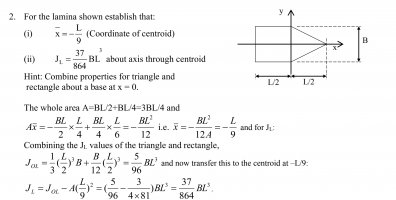2nd Moment of Area Problem

Andywibroe

New member
Hello,

I am going through some old university questions to see if I can still do some of these basics.

In the attached is the problem and the solution I have been looking at. Part (i) is fairly straight forward. However, I cannot follow a part of the process in part (ii) which is what I would appreciate help with.

Firstly, the nomenclature here is JL is the second moment of area about the centroid. I have actually gone through the whole excercise of of numeric integration using Simpson’s rules and do actually arrive at 37/864*B*L^3, however, I cannot follow the given solution. The part I am not following is in the combination of the two second moments of the areas as individual shapes. I concur that the second moment of area of the rectangle about its centroid would be of the form length (L) to the power of 3, multiplied by the breadth (B) over 12 which in this case gives B/12*(L/2)^3 as is in part of the solution. However, the solution gives 1/3*(L/2)^3 *B as the second moment of area for the triangle about its centroid. I am of the understanding that the area of a triangle about its centroid is (L^3*B)/36 which would be (L/2)^3*B/36 which gives L^3*B/288 as opposed to L^3*B/24.

I have also integrated the triangle in isolation using algebraic calculus and Sampson’s rules and in both cases get L^3*B/288 so something is not quite right. When I used numeric integration to get the second moment of area about the centre of the axis system (x=0) I also get 5/96*BL^3 so I do believe the solution provided is correct but I cannot follow the logic as to why. Any thoughts?

Thanks,

Andy.Subhotosh Khan

Super Moderator
Staff member
Hello,

I am going through some old university questions to see if I can still do some of these basics.

In the attached is the problem and the solution I have been looking at. Part (i) is fairly straight forward. However, I cannot follow a part of the process in part (ii) which is what I would appreciate help with.

Firstly, the nomenclature here is JL is the second moment of area about the centroid. I have actually gone through the whole excercise of of numeric integration using Simpson’s rules and do actually arrive at 37/864*B*L^3, however, I cannot follow the given solution. The part I am not following is in the combination of the two second moments of the areas as individual shapes. I concur that the second moment of area of the rectangle about its centroid would be of the form length (L) to the power of 3, multiplied by the breadth (B) over 12 which in this case gives B/12*(L/2)^3 as is in part of the solution. However, the solution gives 1/3*(L/2)^3 *B as the second moment of area for the triangle about its centroid. I am of the understanding that the area of a triangle about its centroid is (L^3*B)/36 which would be (L/2)^3*B/36 which gives L^3*B/288 as opposed to L^3*B/24.

I have also integrated the triangle in isolation using algebraic calculus and Sampson’s rules and in both cases get L^3*B/288 so something is not quite right. When I used numeric integration to get the second moment of area about the centre of the axis system (x=0) I also get 5/96*BL^3 so I do believe the solution provided is correct but I cannot follow the logic as to why. Any thoughts?

Thanks,

Andy.
View attachment 29549
The Area -Moment of the triangle is first calculated about the y-axis.

JOL = 1/36 * B * (L/2)3 + (1/2)*B*(L/2)*(L/6)2 = (B/12) * (L/2)3

The Area -Moment of the rectangle calculated about the y-axis:

JOL = 1/12* B * (L/2)3 + (1)*B*(L/2)*(L/4)2 = (B/3) * (L/2)3

Andywibroe

New member
Thanks,

I see where I’ve misunderstood the given explanation. The fact it says “triangle and square” I assumed that the formula was in that order as the second moment about the y axis for the triangle happens to be the same formula for the second moment of area for the square about its centroid.

many thanks,
Andy.